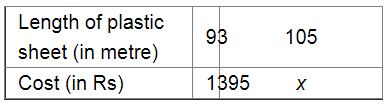# If the cost of 93 m of a certain kind of plastic sheet is Rs 1395,`
Question:

If the cost of 93 m of a certain kind of plastic sheet is Rs 1395, then what would it cost to buy 105 m of such plastic sheet?

Solution:Let the cost of the plastic sheet per metre be Rs $x$.

If more sheets are bought, the cost will also be more.

Therefore, it is a direct variation.

We get:

$93: 105=1395: x$

$\Rightarrow \frac{93}{105}=\frac{1395}{x}$

Applying cross muliplication, we get:

$x=\frac{105 \times 1395}{93}$

$=1575$

Thus, the required cost will be Rs 1,575 .